# HOMER Grid 1.8

 Navigation: HOMER's Calculations How HOMER Calculates Clearness IndexOn the Solar Resource page, for each month of the year, you can enter the average radiation for the month. Based on the value of the average radiation, the month of the year, and the latitude, HOMER calculates the clearness index. This section of the help describes the relationship between the two variables and how HOMER calculates the clearness index from the global horizontal radiation (GHI).

The clearness index is a dimensionless number between zero and one, indicating the fraction of the solar radiation striking the top of the atmosphere that makes it through the atmosphere to strike the Earth's surface. The following equation defines the monthly average clearness index: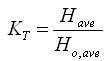where: Have = the monthly average radiation on the horizontal surface of the earth [kWh/m2/day] Ho,ave = the extraterrestrial horizontal radiation, meaning the radiation on a horizontal surface at the top of the earth's atmosphere [kWh/m2/day]

For a given latitude, we can calculate Ho,ave for any month of the year. If you know either Have or KT , HOMER can calculate the other using the above equation every time you enter a value into the monthly data table in the Solar Resource Inputs. If you enter an average radiation value, HOMER calculates the corresponding clearness index.

The following describes how HOMER calculates Ho,ave, the monthly average extraterrestrial radiation.

As described in more detail in the section on calculating the radiation incident on the PV array, HOMER uses the following equation to calculate the intensity of solar radiation at the top of the Earth's atmosphere: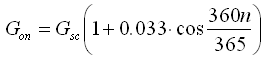where: Gsc = the solar constant [1.367 kW/m2] n = the day of the year [a number between 1 and 365]

The equation above gives the extraterrestrial radiation on a surface normal to the sun's rays. To calculate the extraterrestrial radiation on the horizontal surface, HOMER uses the following equation: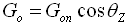where: θZ = the zenith angle [°]

HOMER calculates the zenith angle using the following equation: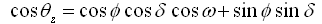where: ϕ = the latitude [°] δ = the solar declination [°] ω = the hour angle [°]

HOMER calculates the solar declination according to the following equation: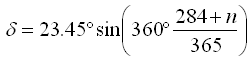where: n = the day of the year [a number between 1 and 365]

We can find the total daily extraterrestrial radiation per square meter by integrating the equation for Go from sunrise to sunset. This integration gives the following equation: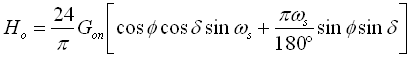where: Ho = the average extraterrestrial horizontal radiation for the day [kWh/m2/day] ωs = the sunset hour angle [°]

HOMER calculates the sunset hour angle using the following equation: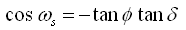HOMER calculates Ho for each day of the month, and finds the average for the month as follows: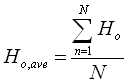where: Ho,ave = the average extraterrestrial horizontal radiation for the month [kWh/m2/day] N = the number of days in the month

If you enter the monthly average global solar radiation, HOMER divides it by Ho,ave to find the monthly average clearness index.